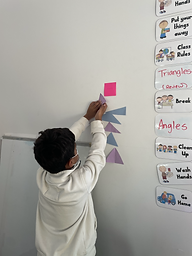## Ms. Caitlyn

### Target 1​

###### Lesson Type:

Continuation

Geometry

:

Shape

Classify 2D and 3D figures based on the basic properties of shapes.

###### 1:

Identify triangles by their defining attributes (number of sides, number of vertices, vertices angle measurements, and side lengths).

###### 2:

Define each type of triangle (scalene, isosceles, equilateral, obtuse, acute, and right).

4th

###### Vocabulary:

Right, Obtuse, Acute, Angle, Scalene, Isosceles, Equilateral, Side

Activities:

Students reviewed identifying triangles by side (scalene, isosceles, equilateral) by sorting them in their respective sections around the room.

Students identified triangles by angle by measuring the angles on each triangle and sorting them in their respective sections around the room.### Home Exploration

###### Guiding Questions:## Absent Students:

### Target 2

:

###### 1:

Accurately measure angles.

###### 2:

Identify angles as acute, obtuse, or right.

###### 3:

Understand that angles are measured in degrees (from 0 to 360).

###### 4:

Understand that angles have types based on their degree measures (acute, obtuse, and right).

5th

###### Vocabulary:

Degree, Angle, Protractor, Acute, Obtuse, Right

Activities:

Students measured the angles of various triangles.

Students measured angles on puzzle pieces from 30 to 180 degrees and matched them to their corresponding measurement.### Home Exploration

###### Guiding Questions:### Target 3

:

###### Vocabulary:

Activities:### Home Exploration